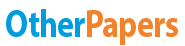# Math for Management Notes

Essay by   •  September 24, 2012  •  Course Note  •  617 Words (3 Pages)  •  1,521 Views

## Essay Preview: Math for Management Notes

Report this essay
Page 1 of 3

Supply and Demand

Measures of Center and Variation

Variance is SD squared

Linear Regression

Probability and Distributions

Suppose an experiment consists of trials, and the probability of success in a given trial is . Let be the random variable that represents the total number of successes in all trials (so, in particular, will take on values from 0 to ). Then the probability that you have exactly successes in trials is

where are known as binomial coefficients, which appear in many places in mathematics.

Suppose a class takes a test, and the results are normally distributed with mean 73 and standard deviation of 15. What is the probability that a random student scored above a 90 or below a 50?

In this question, we're interested in what's going on in the tails of the probability distribution. By the rules of probability distributions, the probability that a student scores above a 90 is one minus the probability the student scores below a 90. Therefore, we should calculate:

Let's say represents the random variable corresponding to the experiment "pick a random student and examine their score." Then recasting in terms of random variables, we want to calculate:

We can convert our -values into -values:

Then our probability is the same as

Central Limit Theorem and Hypothesis Testing

Time Value of Money

Let's summarize the above description of compound interest into a nice formula. If an account is established with dollars at an annual interest rate of (expressed as a decimal between 0 and 1), compounded times per year, then at the end of periods the account will be worth dollars, where

Annuity

Suppose that you purchase a house for \$250,000. You make a down payment of \$50,000, so you finance the balance, \$200,000, over thirty years at an annual interest rate of 6 %. Payments are due monthly, so a total of payments are to be made, at a monthly interest rate of , or .5%.

...

...